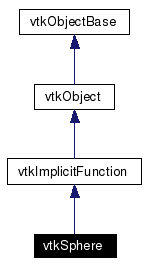# vtkSphere Class Reference

`#include <vtkSphere.h>`

Inheritance diagram for vtkSphere:[legend]
Collaboration diagram for vtkSphere:[legend]
List of all members.

## Detailed Description

implicit function for a sphere

vtkSphere computes the implicit function and/or gradient for a sphere. vtkSphere is a concrete implementation of vtkImplicitFunction.

Examples:
vtkSphere (Examples)
Tests:
vtkSphere (Tests)

Definition at line 33 of file vtkSphere.h.
double EvaluateFunction (double x)
double EvaluateFunction (double x, double y, double z)
static vtkSphereNew ()

## Public Types

typedef vtkImplicitFunction Superclass

## Public Member Functions

virtual const char * GetClassName ()
virtual int IsA (const char *type)
void PrintSelf (ostream &os, vtkIndent indent)
void EvaluateGradient (double x, double n)
virtual void SetRadius (double)
virtual double GetRadius ()
virtual void SetCenter (double, double, double)
virtual void SetCenter (double)
virtual double * GetCenter ()
virtual void GetCenter (double data)

## Static Public Member Functions

static int IsTypeOf (const char *type)
static vtkSphereSafeDownCast (vtkObject *o)

vtkSphere ()
~vtkSphere ()

## Protected Attributes

double Radius
double Center 

## Member Typedef Documentation

 Reimplemented from vtkImplicitFunction. Definition at line 36 of file vtkSphere.h.

## Constructor & Destructor Documentation

 vtkSphere::vtkSphere ( ) ` [protected]`

 vtkSphere::~vtkSphere ( ) ` [inline, protected]`
 Definition at line 66 of file vtkSphere.h.

## Member Function Documentation

 virtual const char* vtkSphere::GetClassName ( ) ` [virtual]`
 Reimplemented from vtkImplicitFunction.

 static int vtkSphere::IsTypeOf ( const char * type ) ` [static]`
 Return 1 if this class type is the same type of (or a subclass of) the named class. Returns 0 otherwise. This method works in combination with vtkTypeRevisionMacro found in vtkSetGet.h. Reimplemented from vtkImplicitFunction.

 virtual int vtkSphere::IsA ( const char * type ) ` [virtual]`
 Return 1 if this class is the same type of (or a subclass of) the named class. Returns 0 otherwise. This method works in combination with vtkTypeRevisionMacro found in vtkSetGet.h. Reimplemented from vtkImplicitFunction.

 static vtkSphere* vtkSphere::SafeDownCast ( vtkObject * o ) ` [static]`
 Reimplemented from vtkImplicitFunction.

 void vtkSphere::PrintSelf ( ostream & os, vtkIndent indent ) ` [virtual]`
 Methods invoked by print to print information about the object including superclasses. Typically not called by the user (use Print() instead) but used in the hierarchical print process to combine the output of several classes. Reimplemented from vtkImplicitFunction.

 static vtkSphere* vtkSphere::New ( ) ` [static]`
 Construct sphere with center at (0,0,0) and radius=0.5. Reimplemented from vtkObject.

 double vtkSphere::EvaluateFunction ( double x ) ` [virtual]`
 Evaluate sphere equation ((x-x0)^2 + (y-y0)^2 + (z-z0)^2) - R^2. Implements vtkImplicitFunction.

 double vtkSphere::EvaluateFunction ( double x, double y, double z ) ` [inline]`
 Evaluate sphere equation ((x-x0)^2 + (y-y0)^2 + (z-z0)^2) - R^2. Reimplemented from vtkImplicitFunction. Definition at line 45 of file vtkSphere.h. References vtkImplicitFunction::EvaluateFunction().

 void vtkSphere::EvaluateGradient ( double x, double n ) ` [virtual]`
 Evaluate sphere gradient. Implements vtkImplicitFunction.

 virtual void vtkSphere::SetRadius ( double ) ` [virtual]`
 Set / get the radius of the sphere.

 virtual double vtkSphere::GetRadius ( ) ` [virtual]`
 Evaluate sphere gradient.

 virtual void vtkSphere::SetCenter ( double , double , double ) ` [virtual]`
 Set / get the center of the sphere.

 virtual void vtkSphere::SetCenter ( double  ) ` [virtual]`
 Set / get the center of the sphere.

 virtual double* vtkSphere::GetCenter ( ) ` [virtual]`
 Set / get the center of the sphere.

 virtual void vtkSphere::GetCenter ( double data ) ` [virtual]`
 Set / get the center of the sphere.

## Member Data Documentation

 double vtkSphere::Radius` [protected]`
 Definition at line 66 of file vtkSphere.h.

 double vtkSphere::Center` [protected]`
 Definition at line 69 of file vtkSphere.h.

The documentation for this class was generated from the following file:

Generated on Tue Jan 22 00:17:28 2008 for VTK by1.4.3-20050530